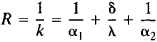Heat Transmission Between Heat-Transfer Fluids

The following article is from The Great Soviet Encyclopedia (1979). It might be outdated or ideologically biased.

Heat Transmission Between Heat-Transfer Fluids

the transmission of heat from one heat-transfer fluid to another through a wall separating the fluids or through an interface between the fluids. Such heat transmission involves the transfer of heat from the hotter fluid to the wall, thermal conduction in the wall, and heat transfer from the wall to the cooler flowing medium.

The intensity of the transmission of heat from one fluid through a wall to another fluid is characterized by the heat-transmission coefficient k, which is the quantity of heat transferred through a wall surface of unit area in unit time when the temperature difference between the fluids is 1°K. The coefficient may be expressed in watts per m2 per degree Kelvin. The inverse R of the heat-transmission coefficient may be called the total thermal resistance. For example, in the case of a single-layer wall,Here, α1 and α2 are the heat-transfer coefficients across the wall surface for the hot fluid and the cold fluid, respectively; δ is the thickness of the wall, and λ is the thermal conductivity of the wall. In practice, the heat-transmission coefficient is generally determined experimentally. The results obtained are processed by the methods of similarity theory.

REFERENCES

Gröber, G., S. Erk, and U. Grigull. Osnovy ucheniia o teploobmene. Moscow, 1958. (Translated from German.)
Shorin, S. N. Teploperedacha, 2nd ed. Moscow, 1964.
Mikheev, M. A., and I. M. Mikheeva. Osnovy teploperedachi, 2nd ed. Moscow, 1973.

I. N. ROZENGAUZ